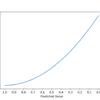# Search results for "regression"## Using autograd in TensorFlow to Solve a Regression Problem

We usually use TensorFlow to build a neural network. However, TensorFlow is not limited to this. Behind the scene, TensorFlow is a tensor library with automatic differentiation capability. Hence we can easily use it to solve a numerical optimization problem with gradient descent. In this post, we are going to show how TensorFlow’s automatic differentiation […]## Neural Network Models for Combined Classification and Regression

Some prediction problems require predicting both numeric values and a class label for the same input. A simple approach is to develop both regression and classification predictive models on the same data and use the models sequentially. An alternative and often more effective approach is to develop a single neural network model that can predict […]## XGBoost for Regression

Extreme Gradient Boosting (XGBoost) is an open-source library that provides an efficient and effective implementation of the gradient boosting algorithm. Shortly after its development and initial release, XGBoost became the go-to method and often the key component in winning solutions for a range of problems in machine learning competitions. Regression predictive modeling problems involve predicting […]## How to Use Optimization Algorithms to Manually Fit Regression Models

Regression models are fit on training data using linear regression and local search optimization algorithms. Models like linear regression and logistic regression are trained by least squares optimization, and this is the most efficient approach to finding coefficients that minimize error for these models. Nevertheless, it is possible to use alternate optimization algorithms to fit […]## Regression Metrics for Machine Learning

Regression refers to predictive modeling problems that involve predicting a numeric value. It is different from classification that involves predicting a class label. Unlike classification, you cannot use classification accuracy to evaluate the predictions made by a regression model. Instead, you must use error metrics specifically designed for evaluating predictions made on regression problems. In […]## Multinomial Logistic Regression With Python

Multinomial logistic regression is an extension of logistic regression that adds native support for multi-class classification problems. Logistic regression, by default, is limited to two-class classification problems. Some extensions like one-vs-rest can allow logistic regression to be used for multi-class classification problems, although they require that the classification problem first be transformed into multiple binary […]## Autoencoder Feature Extraction for Regression

Autoencoder is a type of neural network that can be used to learn a compressed representation of raw data. An autoencoder is composed of encoder and a decoder sub-models. The encoder compresses the input and the decoder attempts to recreate the input from the compressed version provided by the encoder. After training, the encoder model […]## Multivariate Adaptive Regression Splines (MARS) in Python

Multivariate Adaptive Regression Splines, or MARS, is an algorithm for complex non-linear regression problems. The algorithm involves finding a set of simple linear functions that in aggregate result in the best predictive performance. In this way, MARS is a type of ensemble of simple linear functions and can achieve good performance on challenging regression problems […]## How to Develop LARS Regression Models in Python

Regression is a modeling task that involves predicting a numeric value given an input. Linear regression is the standard algorithm for regression that assumes a linear relationship between inputs and the target variable. An extension to linear regression involves adding penalties to the loss function during training that encourage simpler models that have smaller coefficient […]## How to Develop LASSO Regression Models in Python

Regression is a modeling task that involves predicting a numeric value given an input. Linear regression is the standard algorithm for regression that assumes a linear relationship between inputs and the target variable. An extension to linear regression invokes adding penalties to the loss function during training that encourages simpler models that have smaller coefficient […]